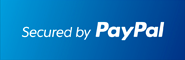** New: ECONOMICS , DIFFERENTIAL EQUATIONS **         ** SPECIALS for bulk apps **         ** Bestsellers: CALCULUS , SAT , PHYSICS , CHEM , STOCKS & PORTFOLIOS , ALGEBRA **# #1 Number Theory Solver for the TI-89 Calculator

## Solve Number Theory problems Step by Step.

### Works With TI92 Plus TI89 TI89 Titanium Voyage 200At the end of the PayPal checkout, you will be sent an email containing your key and software.Description

• The most comprehensive Number Theory APP for calculators.
• Users have boosted their Number Theory knowledge.
• Shows step by step solutions for some Number Theory topics such as RSA Encryption, Euler's Phi Function, Solving Congruences, Euclidean Algorithm, Perfect Numbers, Finding GCD and LCM, Euclid's Theorem on Number of Primes, etc.
• Includes Diophantine Equations Solver, Mersenne, Prime and CoPrime Checker, Extended Euclidean Algorithm, Perfect Numbers. Perform Modular Arithnmetic, Ring vs Field, Find the Order of Integers mod M, Finding Inverse mod M, Primitive Roots, Quadratic Reciprocity, Solving Congruences Ax=B mod M and x^2=A mod M, Compute Legendre Symbol, and more.
• Verify Theorems and Conjectures such as Chinese Remainder Theorem, Fermat's Little and Last Theorem, Euler's Theorem, Goldbach Conjecture, Twin Primes.
• Contains both Theorems, Rules and Computations.
• Also: Step by Step Complex Numbers, Step by Step Synthetic Division, Matrix Computations, Combinatorics (incl. Combinations and Permutations), Sequences, Quadratic Equations (Completing the square - step by step), Solve 2x2 or nxn System of Equations and much more...
• Continued Fractions
• Ideal for quick review and homework check.
• Easy to use. Just plug in and the correct answer shows.

Visit the Classic Site at www.ti89.com/index.htm for more details.

Documentation